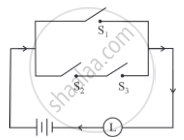# Express the following circuit in the symbolic form of logic and writ the input-output table. - Mathematics and Statistics

Sum

Express the following circuit in the symbolic form of logic and writ the input-output table.#### Solution

Let p: the switch S1 is closed
q: the switch S2 is closed
r: the switch S3 is closed
∼ p: the switch S1′ is closed or the switch S1 is open
∼ q: the switch S2′ is closed or the switch S2 is open
∼ r: the switch S3′ is closed or the switch S3 is open
l: the lamp L is on

The symbolic form of the given circuit is:
p ∨ (q ∧ r) ≡ l
l is generally dropped and it can be expressed as:
p ∨ (q ∧ r)

Input-Output Table

 p q r q ∧ r p ∨ (q ∧ r) 1 1 1 1 1 1 1 0 0 1 1 0 1 0 1 1 0 0 0 1 0 1 1 1 1 0 1 0 0 0 0 0 1 0 0 0 0 0 0 0
Concept: Application of Logic to Switching Circuits
Is there an error in this question or solution?